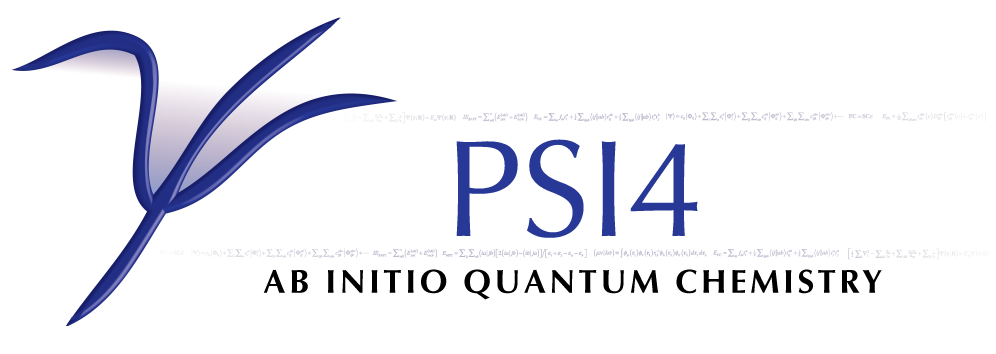# DETCI¶

Performs configuration interaction (CI) computations of various types, including restricted-active-space (RAS) CI, full CI, the CI component of multi-configuration self-consistent-field (MCSCF) and complete-active-space self-consistent-field (CASSCF) computations, and arbitrary-order perturbation theory and arbitrary-order coupled-cluster computations for small molecules.

## General Options¶

### AVG_STATES¶

Array giving the root numbers of the states to average in a state-averaged procedure such as SA-CASSCF. Root numbering starts from 1.

• Type: array
• Default: No Default

### AVG_WEIGHTS¶

Array giving the weights for each state in a state-averaged procedure

• Type: array
• Default: No Default

### CIBLKS_PRINT¶

Do print a summary of the CI blocks?

• Type: integer
• Default: 1

### DETCI_FREEZE_CORE¶

Do freeze core orbitals?

### EX_LEVEL¶

The CI excitation level

• Type: integer
• Default: 2

### E_CONVERGENCE¶

Convergence criterion for energy.

### FCI¶

Do a full CI (FCI)? If TRUE, overrides the value of EX_LEVEL

### ICORE¶

Specifies how to handle buffering of CI vectors. A value of 0 makes the program perform I/O one RAS subblock at a time; 1 uses entire CI vectors at a time; and 2 uses one irrep block at a time. Values of 0 or 2 cause some inefficiency in the I/O (requiring multiple reads of the C vector when constructing H in the iterative subspace if DIAG_METHOD = SEM), but require less core memory.

• Type: integer
• Default: 1

### ISTOP¶

Do stop DETCI after string information is formed and before integrals are read?

### MAXITER¶

Maximum number of iterations to diagonalize the Hamiltonian

• Type: integer
• Default: 12

### NUM_DETS_PRINT¶

Number of important determinants to print

• Type: integer
• Default: 20

### NUM_ROOTS¶

number of CI roots to find

• Type: integer
• Default: 1

### REFERENCE¶

Reference wavefunction type

• Type: string
• Possible Values: RHF, ROHF
• Default: RHF

### R_CONVERGENCE¶

Convergence criterion for CI residual vector in the Davidson algorithm (RMS error). The default is 1e-4 for energies and 1e-7 for gradients.

### S_SQUARED¶

Do calculate the value of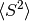for each root?

### VAL_EX_LEVEL¶

In a RAS CI, this is the additional excitation level for allowing electrons out of RAS I into RAS II. The maximum number of holes in RAS I is therefore EX_LEVEL + VAL_EX_LEVEL.

• Type: integer
• Default: 0

## Specifying the CI Space¶

### ACTIVE¶

An array giving the number of active orbitals (occupied plus unoccupied) per irrep (shorthand to make MCSCF easier to specify than using RAS keywords)

• Type: array
• Default: No Default

### A_RAS3_MAX¶

maximum number of alpha electrons in RAS III

• Type: integer
• Default: -1

### B_RAS3_MAX¶

maximum number of beta electrons in RAS III

• Type: integer
• Default: -1

### MS0¶

Do use the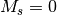component of the state? Defaults to TRUE if closed-shell and FALSE otherwise. Related to the S option.

### RAS34_MAX¶

maximum number of electrons in RAS III + IV

• Type: integer
• Default: -1

### RAS3_MAX¶

maximum number of electrons in RAS III

• Type: integer
• Default: -1

### RAS4_MAX¶

maximum number of electrons in RAS IV

• Type: integer
• Default: -1

### RESTRICTED_DOCC¶

An array giving the number of restricted doubly-occupied orbitals per irrep (not excited in CI wavefunctions, but orbitals can be optimized in MCSCF)

• Type: array
• Default: No Default

### RESTRICTED_UOCC¶

An array giving the number of restricted unoccupied orbitals per irrep (not occupied in CI wavefunctions, but orbitals can be optimized in MCSCF)

• Type: array
• Default: No Default

### S¶

The value of the spin quantum number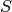is given by this option. The default is determined by the value of the multiplicity. This is used for two things: (1) determining the phase of the redundant half of the CI vector when thecomponent is used (i.e., MS0 = TRUE), and (2) making sure the guess vector has the desired value of(if S_SQUARED is TRUE and ICORE = 1).

• Type: double
• Default: 0.0

## Diagonalization Methods¶

### DIAG_METHOD¶

This specifies which method is to be used in diagonalizing the Hamiltonian. The valid options are: RSP, to form the entire H matrix and diagonalize using libciomr to obtain all eigenvalues (n.b. requires HUGE memory); OLSEN, to use Olsen’s preconditioned inverse subspace method (1990); MITRUSHENKOV, to use a 2x2 Olsen/Davidson method; and DAVIDSON (or SEM) to use Liu’s Simultaneous Expansion Method, which is identical to the Davidson method if only one root is to be found. There also exists a SEM debugging mode, SEMTEST. The SEM method is the most robust, but it also requires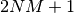CI vectors on disk, where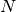is the maximum number of iterations and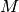is the number of roots.

• Type: string
• Possible Values: RSP, OLSEN, MITRUSHENKOV, DAVIDSON, SEM, SEMTEST
• Default: SEM

### LSE¶

Do use least-squares extrapolation in iterative solution of CI vector?

### LSE_COLLAPSE¶

Number of iterations between least-squares extrapolations

• Type: integer
• Default: 3

### LSE_TOLERANCE¶

Minimum converged energy for least-squares extrapolation to be performed

### PRECONDITIONER¶

This specifies the type of preconditioner to use in the selected diagonalization method. The valid options are: DAVIDSON which approximates the Hamiltonian matrix by the diagonal elements; H0BLOCK_INV which uses an exact Hamiltonian of H0_BLOCKSIZE and explicitly inverts it; GEN_DAVIDSON which does a spectral decomposition of H0BLOCK; ITER_INV using an iterative approach to obtain the correction vector of H0BLOCK. The H0BLOCK_INV, GEN_DAVIDSON, and ITER_INV approaches are all formally equivalent but the ITER_INV is less computationally expensive. Default is DAVIDSON.

• Type: string
• Possible Values: LANCZOS, DAVIDSON, GEN_DAVIDSON, H0BLOCK, H0BLOCK_INV, ITER_INV, H0BLOCK_COUPLING, EVANGELISTI
• Default: DAVIDSON

### UPDATE¶

The update or correction vector formula, either DAVIDSON (default) or OLSEN.

• Type: string
• Possible Values: DAVIDSON, OLSEN
• Default: DAVIDSON

## Density Matrices¶

### DIPMOM¶

Do compute the dipole moment?

### NAT_ORBS_WRITE¶

Do write the natural orbitals?

### NAT_ORBS_WRITE_ROOT¶

Sets the root number for which CI natural orbitals are written to PSIF_CHKPT. The default value is 1 (lowest root).

• Type: integer
• Default: 1

### OPDM¶

Do compute one-particle density matrix if not otherwise required?

### OPDM_AVG¶

Do average the OPDM over several roots in order to obtain a state-average one-particle density matrix? This density matrix can be diagonalized to obtain the CI natural orbitals.

### OPDM_PRINT¶

Do print the one-particle density matrix for each root?

### TDM¶

Do compute the transition density? Note: only transition densities between roots of the same symmetry will be evaluated. DETCI does not compute states of different irreps within the same computation; to do this, lower the symmetry of the computation.

### TDM_PRINT¶

Do print the transition density?

### TDM_WRITE¶

Do write the transition density?

### TPDM¶

Do compute two-particle density matrix if not otherwise required?

### TPDM_PRINT¶

Do print the two-particle density matrix? (Warning: large tensor)

## Root Following¶

### FOLLOW_ROOT¶

The root to write out the two-particle density matrix for (the one-particle density matrices are written for all roots). Useful for a state-specific CASSCF or CI optimization on an excited state.

• Type: integer
• Default: 1

## Guess Vectors¶

### RESTART¶

Do restart a DETCI iteration that terminated prematurely? It assumes that the CI and sigma vectors are on disk; the number of vectors specified by RESTART_VECS (obsolete) is collapsed down to one vector per root.

## File Handling¶

### COLLAPSE_SIZE¶

Gives the number of vectors to retain when the Davidson subspace is collapsed (see MAX_NUM_VECS . If greater than one, the collapsed subspace retains the best estimate of the CI vector for the previous n iterations. Defaults to 1.

• Type: integer
• Default: 1

### MAX_NUM_VECS¶

Maximum number of Davidson subspace vectors which can be held on disk for the CI coefficient and sigma vectors. (There is one H(diag) vector and the number of D vectors is equal to the number of roots). When the number of vectors on disk reaches the value of MAX_NUM_VECS, the Davidson subspace will be collapsed to COLLAPSE_SIZE vectors for each root. This is very helpful for saving disk space. Defaults to MAXITER * NUM_ROOTS + NUM_INIT_VECS

• Type: integer
• Default: 0

### NUM_VECS_WRITE¶

Number of vectors to export

• Type: integer
• Default: 1

### VECS_WRITE¶

Do store converged vector(s) at the end of the run? The vector(s) is(are) stored in a transparent format such that other programs can use it easily. The format is specified in psi4/src/lib/libqt/slaterdset.h .

## General-Order Perturbation Theory¶

### MPN¶

Do compute the MPn series out to kth order where k is determined by MAX_NUM_VECS ? For open-shell systems REFERENCE is ROHF, WFN is ZAPTN), DETCI will compute the ZAPTn series. GUESS_VECTOR must be set to UNIT, HD_OTF must be set to TRUE, and HD_AVG must be set to orb_ener; these should happen by default for MPN = TRUE.

## General-Order Coupled-Cluster¶

### CC¶

Do coupled-cluster computation?

### CC_A_RAS3_MAX¶

maximum number of alpha electrons in RAS III, for CC

• Type: integer
• Default: -1

### CC_B_RAS3_MAX¶

maximum number of beta electrons in RAS III, for CC

• Type: integer
• Default: -1

### CC_EX_LEVEL¶

The CC excitation level

• Type: integer
• Default: 2

### CC_RAS34_MAX¶

maximum number of electrons in RAS III + IV, for CC

• Type: integer
• Default: -1

### CC_RAS3_MAX¶

maximum number of electrons in RAS III, for CC

• Type: integer
• Default: -1

### CC_RAS4_MAX¶

maximum number of electrons in RAS IV, for CC

• Type: integer
• Default: -1

### CC_VAL_EX_LEVEL¶

The CC valence excitation level

• Type: integer
• Default: 0

Do import a CC vector from disk?

### CC_VECS_WRITE¶

Do export a CC vector to disk?

### DIIS¶

Do use DIIS extrapolation to accelerate CC convergence?

### DIIS_FREQ¶

How often to do a DIIS extrapolation. 1 means do DIIS every iteration, 2 is every other iteration, etc.

• Type: integer
• Default: 1

### DIIS_MAX_VECS¶

Maximum number of error vectors stored for DIIS extrapolation

• Type: integer
• Default: 5

### DIIS_MIN_VECS¶

Minimum number of error vectors stored for DIIS extrapolation

• Type: integer
• Default: 2

### DIIS_START_ITER¶

Iteration at which to start using DIIS

• Type: integer
• Default: 1

### NUM_AMPS_PRINT¶

Number of important CC amplitudes per excitation level to print. CC analog to NUM_DETS_PRINT

• Type: integer
• Default: 10

## Expert General Options¶

### SIGMA_OVERLAP¶

Do print the sigma overlap matrix? Not generally useful.

### WFN¶

Wavefunction type. This should be set automatically from the calling Psithon function.

• Type: string
• Possible Values: DETCI, CI, ZAPTN, DETCAS, CASSCF, RASSCF
• Default: DETCI

## Expert Specifying the CI Space¶

### EX_ALLOW¶

An array of length EX_LEVEL specifying whether each excitation type (S,D,T, etc.) is allowed (1 is allowed, 0 is disallowed). Used to specify non-standard CI spaces such as CIST.

• Type: array
• Default: No Default

### MIXED¶

Do allow “mixed” RAS II/RAS III excitations into the CI space? If FALSE, then if there are any electrons in RAS III, then the number of holes in RAS I cannot exceed the given excitation level EX_LEVEL

### MIXED4¶

Do allow “mixed” excitations involving RAS IV into the CI space. Useful to specify a split-virtual CISD[TQ] computation. If FALSE, then if there are any electrons in RAS IV, then the number of holes in RAS I cannot exceed the given excitation level EX_LEVEL

### R4S¶

Do restrict strings with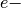in RAS IV? Useful to reduce the number of strings required if MIXED4=true, as in a split-virutal CISD[TQ] computation. If more than one electron is in RAS IV, then the holes in RAS I cannot exceed the number of particles in RAS III + RAS IV (i.e., EX_LEVEL , or else the string is discarded.

### RAS1¶

An array giving the number of orbitals per irrep for RAS1

• Type: array
• Default: No Default

### RAS2¶

An array giving the number of orbitals per irrep for RAS2

• Type: array
• Default: No Default

### RAS3¶

An array giving the number of orbitals per irrep for RAS3

• Type: array
• Default: No Default

### RAS4¶

An array giving the number of orbitals per irrep for RAS4

• Type: array
• Default: No Default

### SF_RESTRICT¶

Do eliminate determinants not valid for spin-complete spin-flip CI’s? [see J. S. Sears et al, J. Chem. Phys. 118, 9084-9094 (2003)]

## Expert Diagonalization Methods¶

### H0_BLOCKSIZE¶

This parameter specifies the size of the H0 block of the Hamiltonian which is solved exactly. The n determinants with the lowest SCF energy are selected, and a submatrix of the Hamiltonian is formed using these determinants. This submatrix is used to accelerate convergence of the CI iterations in the OLSEN and MITRUSHENKOV iteration schemes, and also to find a good starting guess for the SEM method if GUESS_VECTOR is H0_BLOCK. Defaults to 400. Note that the program may change the given size for Ms=0 cases MS0 is TRUE) if it determines that the H0 block includes only one member of a pair of determinants related by time reversal symmetry. For very small block sizes, this could conceivably eliminate the entire H0 block; the program should print warnings if this occurs.

• Type: integer
• Default: 400

### H0_BLOCK_COUPLING¶

Do use coupling block in preconditioner?

### H0_BLOCK_COUPLING_SIZE¶

Parameters which specifies the size of the coupling block within the generalized davidson preconditioner.

• Type: integer
• Default: 0

### H0_GUESS_SIZE¶

size of H0 block for initial guess

• Type: integer
• Default: 400

### HD_AVG¶

How to average H diag energies over spin coupling sets. HD_EXACT uses the exact diagonal energies which results in expansion vectors which break spin symmetry. HD_KAVE averages the diagonal energies over a spin-coupling set yielding spin pure expansion vectors. ORB_ENER employs the sum of orbital energy approximation giving spin pure expansion vectors but usually doubles the number of Davidson iterations. EVANGELISTI uses the sums and differences of orbital energies with the SCF reference energy to produce spin pure expansion vectors. LEININGER approximation which subtracts the one-electron contribution from the orbital energies, multiplies by 0.5, and adds the one-electron contribution back in, producing spin pure expansion vectors and developed by Matt Leininger and works as well as EVANGELISTI.

• Type: string
• Possible Values: EVANGELISTI, HD_EXACT, HD_KAVE, ORB_ENER, LEININGER, Z_KAVE
• Default: EVANGELISTI

## Expert Density Matrices¶

### OPDM_KE¶

Do compute the kinetic energy contribution from the correlated part of the one-particle density matrix?

## Expert Root Following¶

### FOLLOW_VECTOR¶

In following a particular root (see FOLLOW_ROOT , sometimes the root number changes. To follow a root of a particular character, one can specify a list of determinants and their coefficients, and the code will follow the root with the closest overlap. The user specifies arrays containing the absolute alpha string indices (A_i below), absolute beta indices (B_i below), and CI coefficients (C_i below) to form the desired vector. The format is FOLLOW_VECTOR = [ [[A_1, B_1], C_1], [[A_2, B_2], C_2], ...].

• Type: array
• Default: No Default

## Expert Guess Vectors¶

### FILTER_GUESS¶

Do invoke the FILTER_GUESS options that are used to filter out some trial vectors which may not have the appropriate phase convention between two determinants? This is useful to remove, e.g., delta states when a sigma state is desired. The user inputs two determinants (by giving the absolute alpha string number and beta string number for each), and also the desired phase between these two determinants for guesses which are to be kept. FILTER_GUESS = TRUE turns on the filtering routine. Requires additional keywords FILTER_GUESS_DET1 FILTER_GUESS_DET2 and FILTER_GUESS_SIGN

### FILTER_GUESS_DET1¶

Array specifying the absolute alpha string number and beta string number for the first determinant in the filter procedure. (See FILTER_GUESS .

• Type: array
• Default: No Default

### FILTER_GUESS_DET2¶

Array specifying the absolute alpha string number and beta string number for the second determinant in the filter procedure. (See FILTER_GUESS .

• Type: array
• Default: No Default

### FILTER_GUESS_SIGN¶

The required phase (1 or -1) between the two determinants specified by FILTER_GUESS_DET1 and FILTER_GUESS_DET2

• Type: integer
• Default: 1

### FILTER_ZERO_DET¶

If present, the code will try to filter out a particular determinant by setting its CI coefficient to zero. FILTER_ZERO_DET = [alphastr, betastr] specifies the absolute alpha and beta string numbers of the target determinant. This could be useful for trying to exclude states that have a nonzero CI coefficient for the given determinant. However, this option was experimental and may not be effective.

• Type: array
• Default: No Default

### GUESS_VECTOR¶

Guess vector type. Accepted values are UNIT for a unit vector guess NUM_ROOTS and NUM_INIT_VECS must both be 1); H0_BLOCK to use eigenvectors from the H0 BLOCK submatrix (default); DFILE to use NUM_ROOTS previously converged vectors in the D file; IMPORT to import a guess previously exported from a CI computation (possibly using a different CI space)

• Type: string
• Possible Values: UNIT, H0_BLOCK, DFILE, IMPORT
• Default: H0_BLOCK

### NUM_INIT_VECS¶

The number of initial vectors to use in the CI iterative procedure. Defaults to the number of roots.

• Type: integer
• Default: 0

### REFERENCE_SYM¶

Irrep for CI vectors; -1 = find automatically. This option allows the user to look for CI vectors of a different irrep than the reference. This probably only makes sense for Full CI, and it would probably not work with unit vector guesses. Numbering starts from zero for the totally-symmetric irrep.

• Type: integer
• Default: -1

## Expert File Handling¶

### HD_OTF¶

Do compute the diagonal elements of the Hamiltonian matrix on-the-fly? Otherwise, a diagonal element vector is written to a separate file on disk.

### NO_DFILE¶

Do use the last vector space in the BVEC file to write scratch DVEC rather than using a separate DVEC file? (Only possible if NUM_ROOTS = 1.)

## Expert General-Order Perturbation Theory¶

### MPN_ORDER_SAVE¶

If 0, save the MPn energy; if 1, save the MP(2n-1) energy (if available from MPN_WIGNER = true); if 2, save the MP(2n-2) energy (if available from MPN_WIGNER = true).

• Type: integer
• Default: 0

### MPN_SCHMIDT¶

Do employ an orthonormal vector space rather than storing the kth order wavefunction?

### MPN_WIGNER¶

Do use Wigner formulas in the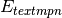series?

### PERTURB_MAGNITUDE¶

The magnitude of perturbation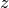in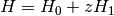• Type: double
• Default: 1.0

## Expert General-Order Coupled-Cluster¶

### CC_FIX_EXTERNAL¶

Do fix amplitudes involving RAS I or RAS IV? Useful in mixed MP2-CC methods.

### CC_FIX_EXTERNAL_MIN¶

Number of external indices before amplitude gets fixed by CC_FIX_EXTERNAL Experimental.

• Type: integer
• Default: 1

### CC_MACRO¶

CC_MACRO = [ [ex_lvl, max_holes_I, max_parts_IV, max_I+IV], [ex_lvl, max_holes_I, max_parts_IV, max_I+IV], ... ] Optional additional restrictions on allowed exictations in coupled-cluster computations, based on macroconfiguration selection. For each sub-array, [ex_lvl, max_holes_I, max_parts_IV, max_I+IV], eliminate cluster amplitudes in which: [the excitation level (holes in I + II) is equal to ex_lvl] AND [there are more than max_holes_I holes in RAS I, there are more than max_parts_IV particles in RAS IV, OR there are more than max_I+IV quasiparticles in RAS I + RAS IV].

• Type: array
• Default: No Default

### CC_MIXED¶

Do ignore block if num holes in RAS I and II is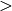cc_ex_lvl and if any indices correspond to RAS I or IV (i.e., include only all-active higher excitations)?

### CC_UPDATE_EPS¶

Do update T amplitudes with orbital eigenvalues? (Usually would do this). Not doing this is experimental.

### CC_VARIATIONAL¶

Do use variational energy expression in CC computation? Experimental.

## Expert Alternative Algorithms¶

### BENDAZZOLI¶

Do use some routines based on the papers of Bendazzoli et al. to calculate sigma? Seems to be slower and not worthwhile; may disappear eventually. Works only for full CI and I don’t remember if I could see how their clever scheme might be extended to RAS in general.

### FCI_STRINGS¶

Do store strings specifically for FCI? (Defaults to TRUE for FCI.)

### REPL_OTF¶

Do string replacements on the fly in DETCI? Can save a gigantic amount of memory (especially for truncated CI’s) but is somewhat flaky and hasn’t been tested for a while. It may work only works for certain classes of RAS calculations. The current code is very slow with this option turned on.